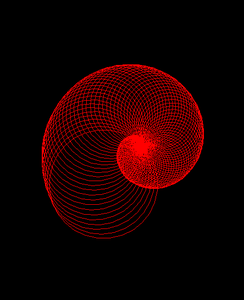Open in App
Not now

# Draw Spiralling Circles Using Turtle Graphics in Python

• Difficulty Level : Medium
• Last Updated : 01 Oct, 2020

Turtle is an inbuilt module of python. It enables us to draw any drawing by a turtle, methods defined in the turtle module, and by using some logical loops. To draw something on the screen(cardboard) just move the turtle(pen). To move turtle(pen) there are some functions i.e forward(), backward(), etc.

Approach:

1. Import and create a turtle instance.
2. Set the graphical visuals as per your needs.
3. Run a for loop for some integer values i.
4. For each value of i, draw a circle with a radius as i.
5. Now rotate the turtle by a fixed degree.

Below is the implementation of the above approach

## Python3

 `# importing turtle``import` `turtle`` ` `# initialise the turtle instance``animation ``=` `turtle.Turtle()`` ` `#creating animation``# changes speed of turtle``animation.speed(``0``)`` ` `# hiding turtle ``animation.hideturtle()`` ` `# changing background color``animation.getscreen().bgcolor(``"black"``)`` ` `# color of the animation``animation.color(``"red"``)`` ` `for` `i ``in` `range``(``100``):``     ` `    ``# drawing circle using circle function ``    ``# by passing radius i``    ``animation.circle(i)`` ` `    ``# changing turtle face by 5 degree from it's``    ``# previous position after drawing a circle``    ``animation._rotate(``5``)`

Output:My Personal Notes arrow_drop_up